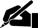# Term Paper: Financial Development and Economic Growth

Pages: 7 (2291 words)  ·  Bibliography Sources: 1+  ·  Level: College Senior  ·  Topic: Economics  ·  Buy This Paper

SAMPLE EXCERPT:

[. . .] We will then examine as to what extent the development in the financial markets of both the countries have contributed to their economic growth. To measure the effect of financial development, we will make use of regression analysis by assuming that economic development is directly related to the financial development of an economy or in other words economic growth is dependent on the financial development of any country.

To analyze the relation of economic growth with financial development, we will measure the increase or decrease in GDP of both the countries with the increase or decrease of their stock market size (or investment in stock market). Even though, GDP and stock market size of any economy are not the sole indicator of its economic or financial performance but it is not possible to compare the entire related factor and the effects in their increases or decrease. To make our analysis simple we are assuming that all other factors are constant.

In case of United States, we have taken the figures for the years 1901 to 1910 (as shown in the table 1)

Table 1:

Stock Market size (in millions of dollars)

GDP (in millions of dollars)

Similarly, in case of Germany, we can analyze the economic growth by comparing the two factors i.e. stock market size and GDP as shown in table # 2.

Table 2:

Stock Market size (in millions of marks)

GDP (in millions of marks)

As discussed before, we will be assuming that the economic growth (or the growth in GDP) is directly related to the growth in financial markets (in our case only stock market); we can state this assumption as:

ax + b

Where y = GDP and x = stock market size

Conclusion:

By plotting the above mentioned figures on a graph, we are able to measure the relationship of both the factors using the graphical representation as shown in the Appendix A. From the figures obtained, it is clear that the increase or decrease in the stock market size affects the GDP and results in the form of its increase or decrease. This reveals that the economic growth is related to the financial development of any country. However, the graphical figures are not in the form of a straight line showing a deviation which indicates that there are some other factors also involved that contribute to the economic development of a country. In case of a situation where financial development might have a direct influence on the economic growth, the graph may have been in the form of the figures shown in Appendix B. The value of a (the vertical intercept) and b (the slope) to obtain the regression line has been calculated as follows:

Stock Market size (in millions of dollars)

GDP (in millions of dollars)

Y

X-X') (Y-Y')

X' = 4281.636

Y' = 26044.27

Therefore, b' = Sum (Xt - X') (Yt - Y') / Sum (Xt - X')2 or b' = 1266680143/6889877 or b' = 183.84

Similarly, a' = Y' - b'X' or a' = 26044.7 - (183.4) 4281.636 or a' = -52480.3

Thus, the equation for regression line thus formed will be'

Y't = - 52480.3 + 183.4X

Similarly, while calculating the values for regression line for determining the relationship in case of Germany, we will compute the solution as follows:

Stock Market size (in millions of dollars)

GDP (in millions of dollars)

Y

X-X') (Y-Y')

X' = 41562.53

And Y' = 5562.727

Therefore, b' = Sum (Xt - X') (Yt - Y') / Sum (Xt - X')2 or b' = 2595999620/324681219.7 or b' = 7.995

Similarly, a' = Y' - b'X' or a' = 5562.727 - (7.995) 41562.53 or a' = -326729.673

Thus, the equation for regression line thus formed will be'

Y't = -326729.673+ 7.995X

Moreover, it is also obvious from the data mentioned above that the United States has experienced a higher growth rate with respect to its increase in market size and which in turn has given rise to an increased growth in GDP. This further validates the point that the financial development or growth of any country has an influence on its economic growth.

From the above mentioned discussion, we came to the conclusion that the presence of a strong and stable financial system in any country not only accumulates capital in the economy but also enhances the economic growth of the country. In case of the United States, the country has a very strong financial system while Germany has a less stable financial system because it has experienced some serious crisis in the financial sector in the past.

References

1) Levine, R (1997),Financial Development and Economic… [END OF PREVIEW]

### Ordering Options:

1.  Download Full Paper (7 Pages)

or

2.  Let us write a NEW paper for you!Most popular!

#### Economics of Peru Thesis…

Cite This Term Paper:

APA Format

Financial Development and Economic Growth.  (2002, April 7).  Retrieved August 22, 2019, from https://www.essaytown.com/subjects/paper/financial-development-economic-growth/444666

MLA Format

"Financial Development and Economic Growth."  7 April 2002.  Web.  22 August 2019. <https://www.essaytown.com/subjects/paper/financial-development-economic-growth/444666>.

Chicago Format

"Financial Development and Economic Growth."  Essaytown.com.  April 7, 2002.  Accessed August 22, 2019.
https://www.essaytown.com/subjects/paper/financial-development-economic-growth/444666.